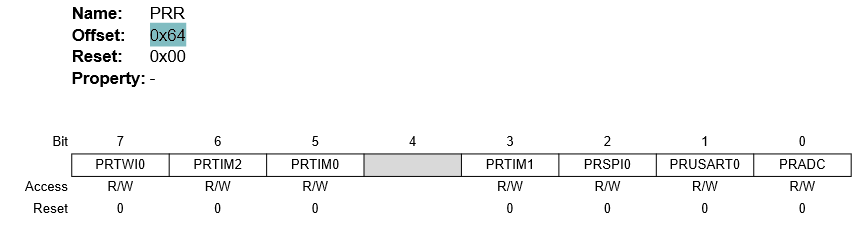Posted by

In this blog, I will discuss minimal programming required for ADC block . This may help embedded beginners to understand implementation of ADC in  Arduino Board using C.

This is in continuation with my last blog post.  I strongly recommend you to read earlier blogs for better understanding . If you find something missing, Please provide feedback in comment box so that I can take corrective action#### Step 1: Make sure  ADC is not shut down#### Step 2: Select reference voltage = AVcc(5V) and required analog pin from multiplexer

• Select VRef  . The ADC count corresponding to VRef is 0x3FF (1023)• Voltage reference and input channel selections will not take effect until ADEN is set

#### Step 4: Start ADC conversion and wait for its completion

• A single conversion is started  by setting PRADC=0 and ADC Start Conversion bit, ADSC=1. ADSC will be cleared by hardware when the conversion is completed.

### Tested Code

//Define required pins and important registers

int pin_A0=14;
int pin_A1=15;

#define mPRR            (*(volatile uint8_t *)0x64)
#define bitVal(bitNum)   1 << bitNum
#define VREF_DEFAULT   0x01

//We are using Serial and PinMode initialization from library. You may refer to earlier blogs //about these . Therefore , In this blog only ADC part will be explained

void setup() {
Serial.begin(115200);
pinMode(LED_BUILTIN, OUTPUT);
pinMode(pin_A0, INPUT);
pinMode(pin_A1, INPUT);
}

float analogPin(uint8_t chNo)
{
unsigned int data,tmpH,tmpL;
float resultV;
data=0;

//Select VREF=01 default Vcc(5V) channel number
mADMUX= (VREF_DEFAULT <<6 ) | (chNo);

//Taking  100 sample reading just for experiment
for(int i=0;i<100;i++)
{

//wait for ADSC flag to be cleared by hardware
data=data+((tmpH << 8)| (tmpL));
delay(10);
}

//Calculate Analog voltage corresponding to data            resultV= (avgADC*5)/1024;
return resultV;
}

void loop() {
Serial.print(“Input voltage at A0=”);
Serial.println(analogPin(0));
delay(5000);
Serial.print(“Input voltage at A1=”);
Serial.println(analogPin(1));

}

### Output

I connected Pin14(A0) to ground and Pin15(A1) to a resister divider voltage=1.72V  measured by Multimeter. Following is the giff file of outputThis is discussed in this video.

References : All documents are available. I am referring to most of these :